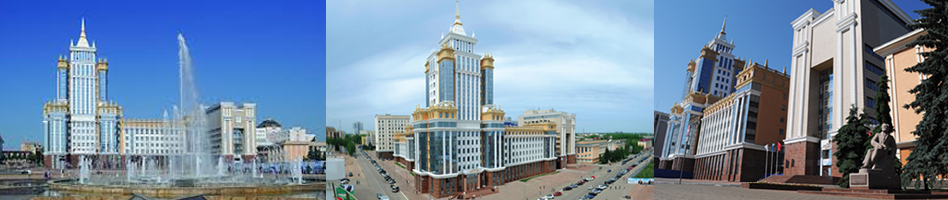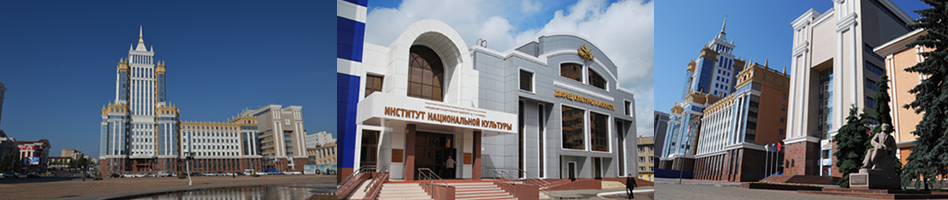To download article.

UDK 004.272.34

DOI: 10.15507/2658-4123.029.201902.187-204

The Multiplication Method with Scaling the Result for High-Precision Residue Positional Interval Logarithmic Computations

Anastasia S. Korzhavina
Senior Lecturer, Chair of Electronic Computing Machines, Vyatka State University (36 Moskovskaya St., Kirov 610000, Russia), ResearcherID: S-1877-2018, ORCID: https://orcid.org/0000-0001-8270-2097, This email address is being protected from spambots. You need JavaScript enabled to view it.

Chief Researcher, Research Institute of Applied and Fundamental Research, Penza State University (40 Krasnaya St., Penza 440026, Russia); Professor, Chair of Electronic Computing Machines, Vyatka State University (36 Moskovskaya St., Kirov 610000, Russia), D.Sc. (Engineering), ResearcherID: Т-4089-2018, ORCID: https://orcid.org/0000-0003-3820-6541, This email address is being protected from spambots. You need JavaScript enabled to view it.

Introduction. The solution of the simulation problems critical to rounding errors, including the problems of computational mathematics, mathematical physics, optimal control, biochemistry, quantum mechanics, mathematical programming and cryptography, requires the accuracy from 100 to 1 000 decimal digits and more. The main lack of high-precision software libraries is a significant decrease of the speed-in-action, unacceptable for practical problems, in particular, when performing multiplication. A way to increase computation performance over very long numbers is using the residue number system. In this work, we discuss a new fast multiplication method with scaling the result using original hybrid residue positional interval logarithmic floating-point number representation.
Materials and Methods. The new way of the organizing numerical information is a residue positional interval logarithmic number representation in which the mantissa is presented in the residue number system, and information on an absolute value (the characteristic) in the interval logarithmic number system that makes it possible to accelerate performance of comparison and scaling is developed to increase the speed of calculations; to compare modular numbers, the provisions of interval analysis are used; to scale modular numbers, the properties of the logarithmic number system and approximate interval calculations using the Chinese reminder theorem are used.
Results. A new fast multiplication method of floating-point residue-represented numbers is developed and patented; the authors evaluated the developed method speed-in action, compared the developed method with classical and pipelined multiplication methods of long numbers.
Discussion and Conclusion. The developed method is 2.4–4.0 times faster than the pipelined multiplication method, and is 6.4–12.9 times faster than classical multiplication methods.

Keywords: residue number system, high-precision computations, multiplication, scaling, interval arithmetic, comparison, logarithmic number system

Funding: The study was funded by the Russian Foundation for Basic Research (project No. 18-37-00278).

For citation: Korzhavina A.S., Knyazkov V.S. The Multiplication Method with Scaling the Result for High-Precision Residue Positional Interval Logarithmic Computations. Inzhenernyye tekhnologii i sistemy = Engineering Technologies and Systems. 2019; 29(2):187-204. DOI: https://doi.org/10.15507/2658-4123.029.201902.187-204

Contribution of the authors: A. S. Korzhavina – reviewing literature, developing the method and analyzing the results. V. S. Knyazkov – formulating the problem, scientific advising, discussing the results.

All authors have read and approved the final version of the paper.

Received 07.12.2018; revised 20.02.2019; published online 28.06.2019

REFERENCES

1. Iakymchuk R., Defour D., Collange S., Graillat S. Reproducible and accurate matrix multiplication. In: Nehmeier M., Wolff von Gudenberg J., Tucker W. (eds) Scientific Computing, Computer Arithmetic and Validated Numerics. SCAN 2015. Lecture Notes in Computer Science. 2016; 9553:126-137.

2. Voros A. Voros A. Discretized Keiper/Li approach to the Riemann hypothesis. Experimental Mathematics. 2018; 1-18.

3. Yang L., Ma D., Ebrahim A., Lloyd C.J., Saunders M.A., Palsson B.O. solveME: fast and reliable solution of nonlinear ME models. BMC Bioinformatics. 2016; 17:391.

4. Panzer E. Algorithms for the symbolic integration of hyperlogarithms with applications to Feynman integrals. Computer Physics Communications. 2015; 188:148-166.

5. Miltenberger M., Ralphs T., Steffy D. E. Exploring the numerics of branch-and-cut for mixed integer linear optimization. In: Kliewer N., Ehmke J., Borndörfer R. (eds) Operations Research Proceedings 2017. Operations Research Proceedings (GOR (Gesellschaft für Operations Research e.V.)). Cham: Springer; 2018. p. 151-157.

6. Bailey D.H., Borwein J.M., Kimberley J.S., Ladd W. Computer discovery and analysis of large poisson polynomials. Experimental Mathematics. 2017; 26(3):349-363.

7. Pan B., Wang Y., Tian S. A high-precision single shooting method for solving hypersensitive optimal control problems. Mathematical Problems in Engineering. 2018; 2018:7908378.

8. Isupov K., Knyazkov V. Interval estimation of relative values in residue number system. Journal of Circuits, Systems and Computers. 2018; 27(1):1850004.

9. Nakasato N., Daisaka H., Fukushige T., Kawai A., Makino J., Ishikawa T., et al. GRAPE-MPs: Implementation of an SIMD for quadruple/hexuple/octuple-precision arithmetic operation on a structured ASIC and an FPGA. In: 2012 IEEE 6th International Symposium on Embedded Multicore SoCs. IEEE; 2012. p. 75-83.

10. Daisaka H., Nakasato N., Ishikawa T., Yuasa F. Application of GRAPE9-MPX for high precision calculation in particle physics and performance results. Procedia Computer Science. 2015; 51:1323-1332.

11. El-Araby E., Gonzalez I., El-Ghazawi T. A. Bringing high-performance reconfigurable computing to exact computations. In: Bertels K., Najjar W., van Genderen A., Vassiliadis S. (eds.) 2007 International Conference on Field Programmable Logic and Applications. 2007. p. 79–85.

12. Lei Y., Dou Y., Zhou J. FPGA-specific custom VLIW architecture for arbitrary precision floatingpoint arithmetic. IEICE Transactions on Information and Systems. 2011; 94(11):2173-2183.

13. Ishii M., Detrey J., Gaudry P., Inomata A., Fujikawa K. Fast modular arithmetic on the Kalray MPPA-256 processor for an energy-efficient implementation of ECM. IEEE Transactions on Computers. 2017; 66(12):2019-2030.

14. Schulte M.J., Swartzlander E.E. A family of variable-precision interval arithmetic processors. IEEE Transactions on Computers. 2000; 49(5):387-397.

15. Asif S., Kong Y. Highly parallel modular multiplier for elliptic curve cryptography in residue number system. Circuits, Systems, and Signal Processing. 2017; 36(3):1027-1051.

16. Kong Y., Lai Y. Low latency modular multiplication for public-key cryptosystems using a scalable array of parallel processing elements. In: 2013 IEEE 56th International Midwest Symposium on Circuits and Systems (MWSCAS). IEEE; 2013. p. 1039-1042.

17. Coleman J.N., Che Ismail R. LNS with co-transformation competes with floating-point. IEEE Transactions on Computers. 2016; 65(1):136-146.

18. Kouretas I., Basetas C., Paliouras V. Low-power logarithmic number system addition/subtraction and their impact on digital filters. IEEE Transactions on Computers. 2013; 62(11):2196-2209.

19. Coleman J.N., Softley C.I., Kadlec J., Matousek R., Tichy M., Pohlet Z., et al. The European logarithmic microprocessor. IEEE Transactions on Computers. 2008; 57(4):532-546.

20. Bigou K., Tisserand A. Single base modular multiplication for efficient hardware RNS implementations of ECC. In: Güneysu T., Handschuh H. (eds) Cryptographic Hardware and Embedded Systems – CHES 2015. Lecture Notes in Computer Science. 2015; 9293:123-140.

21. Bajard J.-C., Eynard J., Merkiche N. Montgomery reduction within the context of residue number system arithmetic. Journal of Cryptographic Engineering. 2018; 8(3):189-200.

22. Czyżak M., Smyk R., Ulman Z. Pipelined scaling of signed residue numbers with the mixedradix conversion in the programmable gate array. Poznan University of Technology Academic Journals. Electrical Engineering. 2013; 76:89-99.

23. Asif S., Hossain M.S., Kong Y., Abdul W. A fully RNS based ECC processor. Integration. 2018; 61:138-149.

24. Matutino P. M., Araújo J., Sousa L., Chaves R. Pipelined FPGA coprocessor for elliptic curve cryptography based on residue number system. In: Patt Y., Nandy S.K. (eds.) 2017 International Conference on Embedded Computer Systems: Architectures, Modeling, and Simulation (SAMOS). 2017. p. 261-268.

25. Korzhavina A.S., Knyazkov V.S. Even faster integer multiplication. Journal of Complexity. 2016; 36:1-30.

26. Harvey D., van der Hoeven J., Lecerf G. Even faster integer multiplication // Journal of Complexity. 2016. Vol. 36. P. 1–30.

27. Chang C.H., Low J.Y.S. Simple, fast, and exact RNS scaler for the three moduli set (2n – 1, 2n, 2n + 1). IEEE Transactions on Circuits and Systems I: Regular Papers. 2011; 58(11):2686-2697.

28. Hiasat A. Efficient RNS scalers for the extended three-moduli set (2n – 1, 2n + p, 2n + 1). IEEE Transactions on Computers. 2017; 66(7):1253-1260.

29. Kong Y., Phillips B. Fast scaling in the residue number system. IEEE Transactions on Very Large Scale Integration (VLSI) Systems. 2009; 17(3):443-447.

30. Meyer-Base U., Stouraitis T. New power-of-2 RNS scaling scheme for cellbased IC design. IEEE Transactions on Very Large Scale Integration (VLSI) Systems. 2003; 11(2):280-283.

31. Johansson F. Arb: Efficient arbitrary-precision midpoint-radius interval arithmetic. IEEE Transactions on Computers. 2017; 66(8):1281-1292.

32. Revol N. Introduction to the IEEE 1788-2015 standard for interval arithmetic. In: Abate A., Boldo S. (eds.) Numerical Software Verification. NSV 2017. Lecture Notes in Computer Science. 2017; 10381:14-21.

33. Osinin I. A modular-logarithmic coprocessor concept. In: International Conference on High Performance Computing & Simulation (HPCS). IEEE. 2017. p. 588-594.

34. Knyazkov V.S., Korzhavina A.S., inventors. The method of organization of multiplying operation of two numbers in floating-point modular-logarithmic format on hybrid multicore processors. Ru Patent 2666285. 2018 Sep 06. (In Russ.)